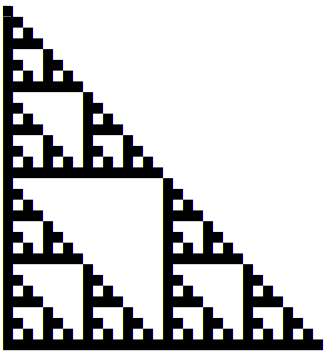# Binary surprise

As mentioned in the previous post, the Gauss-Wantzel theorem says you can construct a regular n-gon with a straight edge and compass if and only if n has the form 2k F where k is a non-negative integer and F is a product of distinct Fermat primes. Let’s look at the binary representation of these values of n.

The 2k factor means that when written in binary, n ends in k zeros.

The only known Fermat primes are 3, 5, 17, 257, and 65537. This means that as far as we know, there are only 25 = 32 possible values for F: each of the five known Fermat primes is either a factor of F or not .

Here are the binary values of the possible values of F.

```1
11
101
1111
10001
110011
1010101
11111111
100000001
1100000011
10100000101
111100001111
1000100010001
11001100110011
101010101010101
1111111111111111
10000000000000001
110000000000000011
1010000000000000101
11110000000000001111
100010000000000010001
1100110000000000110011
10101010000000001010101
111111110000000011111111
1000000010000000100000001
11000000110000001100000011
101000001010000010100000101
1111000011110000111100001111
10001000100010001000100010001
110011001100110011001100110011
1010101010101010101010101010101
11111111111111111111111111111111
```

If you squint, you can see a pattern that looks sorta like a Sierpinski triangle. To make it easier to see, here’s a image of the numbers with the 1’s replaced with black blocks and the 0’s replaced with white space.It’s not just like a Sierpinski triangle, it is a Sierpinski triangle!

## Python code

Here’s Python code to print the binary numbers above.

```from itertools import chain, combinations
from functools import reduce

def product(lst):
return reduce(lambda x,y: x*y, lst, 1)

def powerset(iterable):
xs = list(iterable)
return chain.from_iterable(
combinations(xs,n) for n in range(len(xs)+1)
)

N = 5
# The first N Fermat numbers
F = [2**(2**i)+1 for i in range(N)]

s = {product(x) for x in powerset(F)}
for f in sorted(s):
print(format(f, 'b'))
```

You can continue the pattern by taking all products of subsets of the first few Fermat numbers, but only the first five Fermat numbers are Fermat primes . If you set N to 6 in the code above, for example, you’ll get a triangle with 64 rows.

## More on Sierpinskyi

At first the Sierpinksi triangle seems a fun but someone arbitrary exercise: what happens if I take the middle triangle out of a larger triangle and keep doing that recursively on what’s left? But then it comes up in unexpected ways. I certainly didn’t expect it when I printed out the binary numbers above.

Here are a couple other blog posts where there Sierpinski triangle pops up: one on cellular automata and one on the chaos game.

***

 We allow the possibility that we could have an empty product of Fermat primes, which we interpret at 1. You could think of it this way: each Fermat prime either has an exponent of 0 or 1, and we allow the possibility that all exponents are 0.

 As far as we know. The next several Fermat numbers are definitely not prime, but nobody has proved that there isn’t another Fermat number past 65537 that’s prime.

## 2 thoughts on “Binary surprise”

1. Wow. Why is this true?